# Learning to protect communications with adversarial neural cryptography

Learning to protect communications with adversarial neural cryptography Abadi & Anderson, arXiv 2016

This paper manages to be both tremendous fun and quite thought-provoking at the same time. If I tell you that the central cast contains Alice, Bob, and Eve, you can probably already guess that we’re going to be talking about cryptography (that or reading the paper title 😉 ). But this isn’t cryptography as you know it, and nor is it cryptography intended to actually be used to protect any information – to criticise the paper on that front would be to miss the point in my view. Instead what we get is a really interesting twist on adversarial network training and a further demonstration of the kinds of things that such networks are able to learn.

Here’s the basic setup: there are three neural networks, let’s call them Alice, Bob, and Eve. Alice is given an n-bit message (encoded as a vector of -1.0 or 1.0 values to represent 0 and 1 respectively), and a similarly encoded n-bit key. The job of the Alice network is to produce an n-bit output, which we will call the ciphertext.

Alice’s network has a fully connected layer that is able to mix the message and key together, followed by four 1-dimensional convolutional layers which together reduce the inputs down to an n-bit ciphertext output.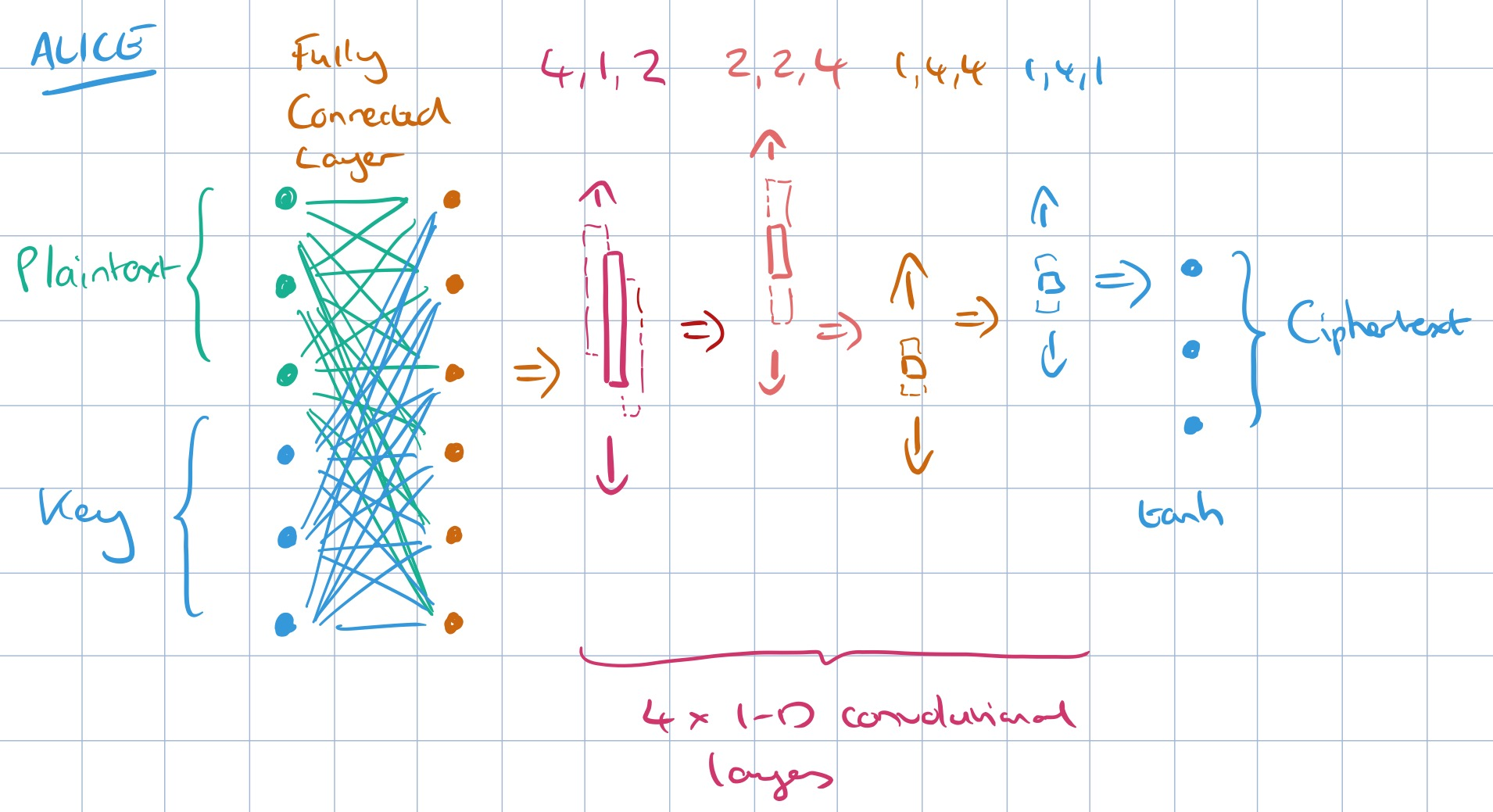The FC layer is followed by a sequence of convolutional layers, the last of which produces an output of a size suitable for a plaintext or ciphertext. These convolutional layers learn to apply some function to groups of the bits mixed by the previous layer, without an a priori specification of what that function should be. Notably, the opposite order (convolutional followed by FC) is much more common in image-processing applications. Neural
networks developed for those applications frequently use convolutions to take advantage of spatial locality. For neural cryptography, we specifically wanted locality—i.e., which bits to combine—to be a learned property, instead of a pre-specified one.

The Bob network receives as input the ciphertext, and same key as was given to Alice. The job of the Bob network is to recover the original message. (Alice and Bob don’t really know that their job is to encode and decode the message, but we can connect the two networks together and use a loss function incorporating the reconstruction error. The structure of Bob’s network is identical to Alice’s: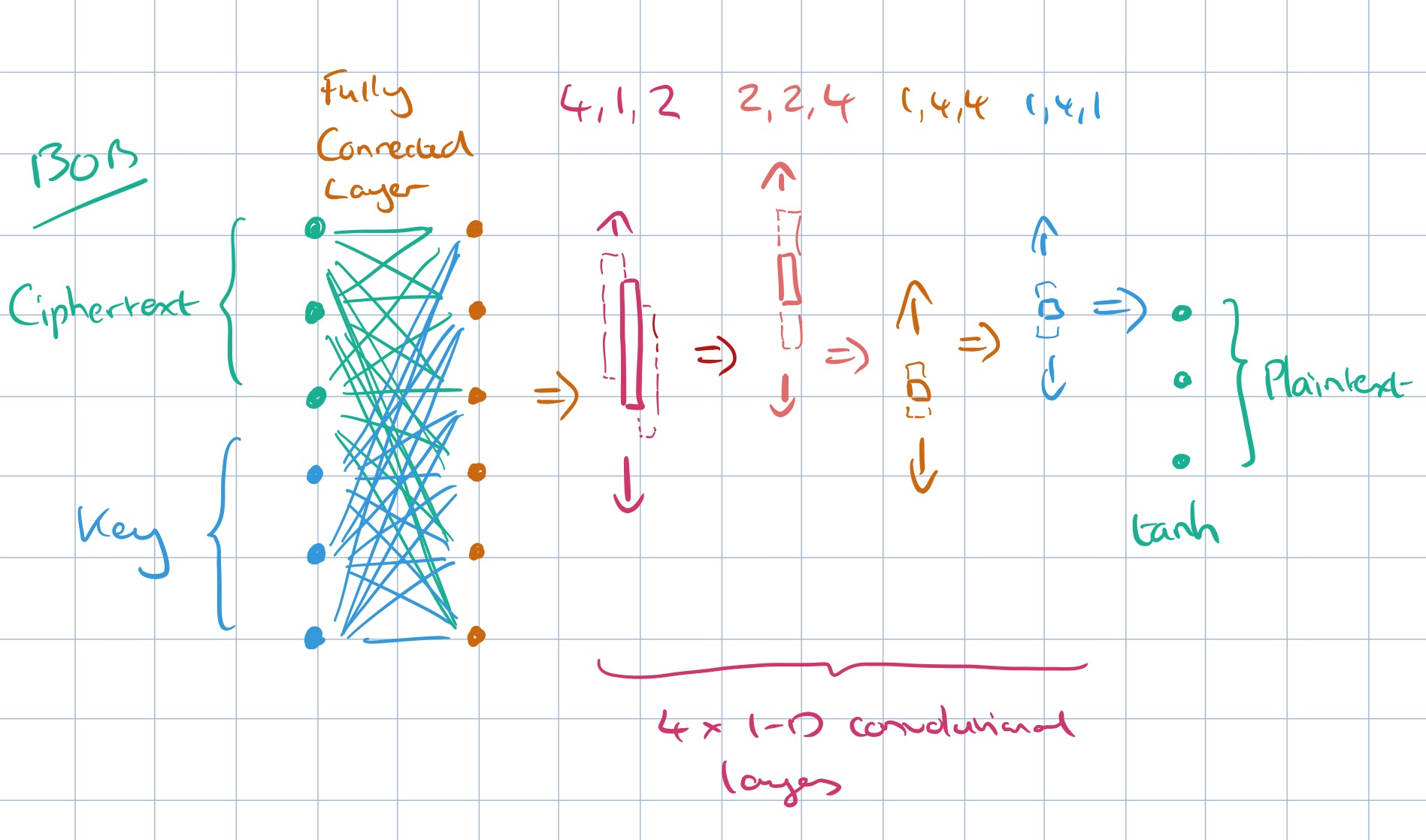If this is all we did, Alice and Bob would quickly learn to ignore the key inputs and just pass the original message through. But now we get to introduce the adversarial member of the cast: Eve. Eve the eavesdropper is also a neural network, but Eve only gets the ciphertext as input, and not the key.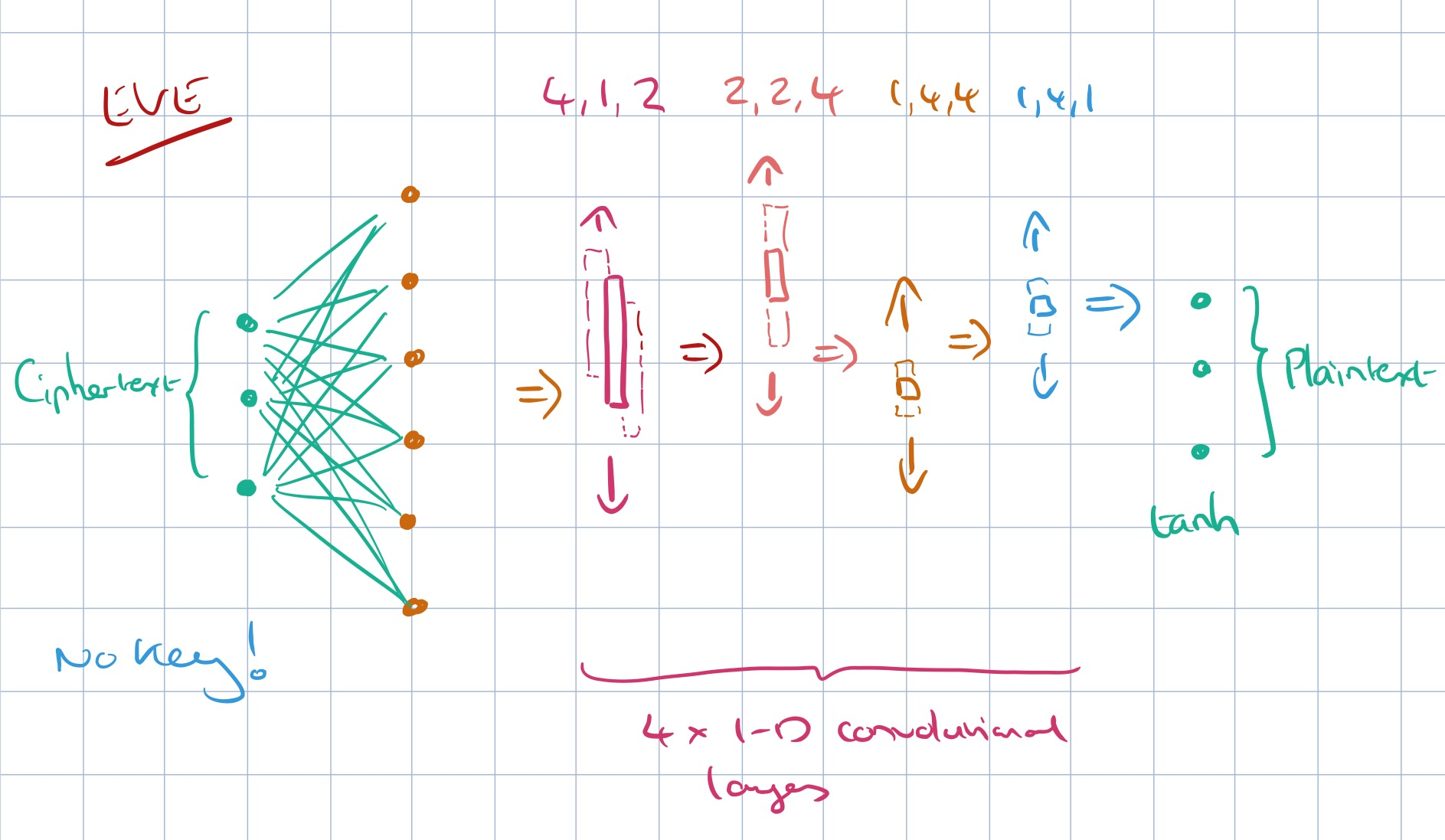Eve’s loss function is setup as the L1 distance from the original message – in other words, Eve is being trained to reconstruct the message from the ciphertext.

The loss function for Alice and Bob is updated so that it also takes into account Eve’s reconstruction score (the better the job Eve does in recovering the message, the larger Alice and Bob’s loss).

The whole thing put together looks like this:Training begins with the networks initialised randomly.

We do not compute the “optimal Eve” for a given value of θA, but simply approximate it, alternating the training of Eve with that of Alice and Bob. Intuitively, the training may for example proceed roughly as follows. Alice may initially produce ciphertexts that neither Bob nor Eve understand at all. By training for a few steps, Alice and Bob may discover a way to communicate that allows Bob to decrypt Alice’s ciphertexts at least partly, but which is not understood by (the present version of) Eve… After a bit of training, however, Eve may start to break this code. With some more training, Alice and Bob may discover refinements, in particular codes that exploit the key material better. Eve eventually finds it impossible to adjust to those codes.

Once training of Alice and Bob has stopped, many instances of Eve are further trained to try and break the cryptosystem.

Now isn’t this a beautiful chart: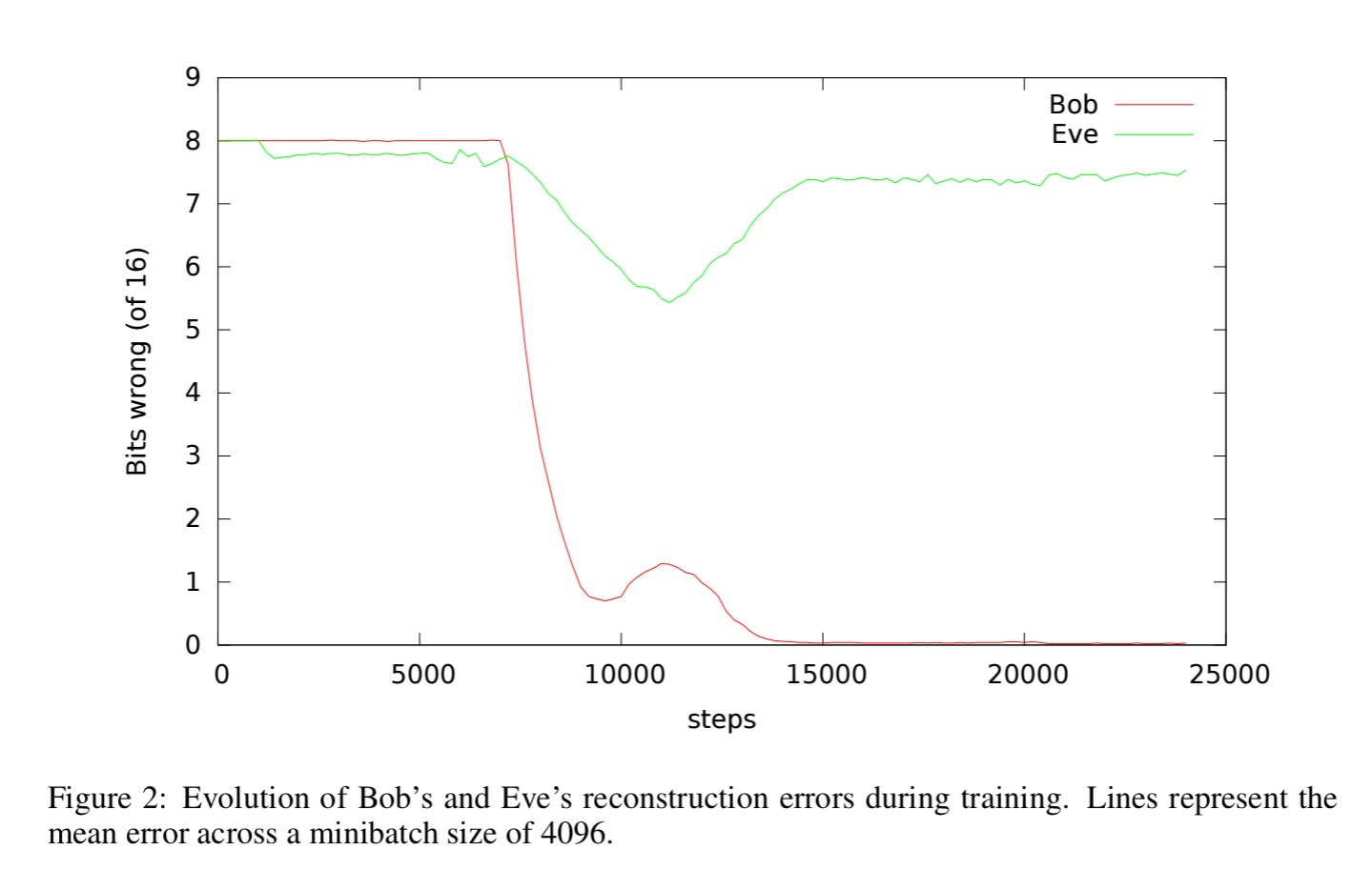The red line traces Bob’s reconstruction error, and the green line is Eve’s. You can see that somewhere around 8000 training steps both Bob and Eve start to be able to reconstruct the original message (though Bob does better than Eve). Somewhere around 10,000 training steps the Alice and Bob networks seem to figure this out and Eve’s error rate climbs again. By 15,000 training steps the training goals are effectively achieved.

This training graph does not look like a typical result for neural networks, where monotonicity in the number of steps is generally expected. Instead, the dynamics of this adversarial training appear somewhat more reminiscent of evolutionary processes. These dynamics appear to depend on somewhat random-seeming change to cause the bits to mix slightly, but once there is some mixing, the gradient descent can rapidly drive it farther.

Manual inspection of one of the trained Alice networks showed that the effect of changing a key bit or a plaintext bit was spread across multiple elements in the ciphertext (and not constrained to a single bit as it would be with an XOR). Typically a one-bit change significantly impacted 3-6 elements in the output, with smaller changes in other elements.

### A refinement: selective protection

We consider the question of whether neural networks can learn what information to protect, given confidentiality objectives described in terms of an adversary.

A dataset is artificially constructed with four-tuples (A,B,C,D). It is loosely inspired by real-world tasks such as having attributes of a user A,B,C, and using them to make a prediction of D. The objective is to make this prediction as accurate as possible, without revealing any information that would allow C to be recovered (i.e., C is the confidential information we want to protect).

The adversary Eve is trained to minimise loss when recovering C from the ciphertext and public prediction of D that are the outputs of Alice. Bob has the ciphertext, public prediction of D, and of course the key shared with Alice, it is Bob’s job to recover the true value of D as closely as possible. The loss function for Alice and Bob is based on how close to the true D the outputs of both Alice and Bob are, as well has how close to the true C Eve is able to get.

Update: in the diagram below the ‘Alice’ in red on the right-hand side should say ‘Eve’. Thanks to Ron for the catch.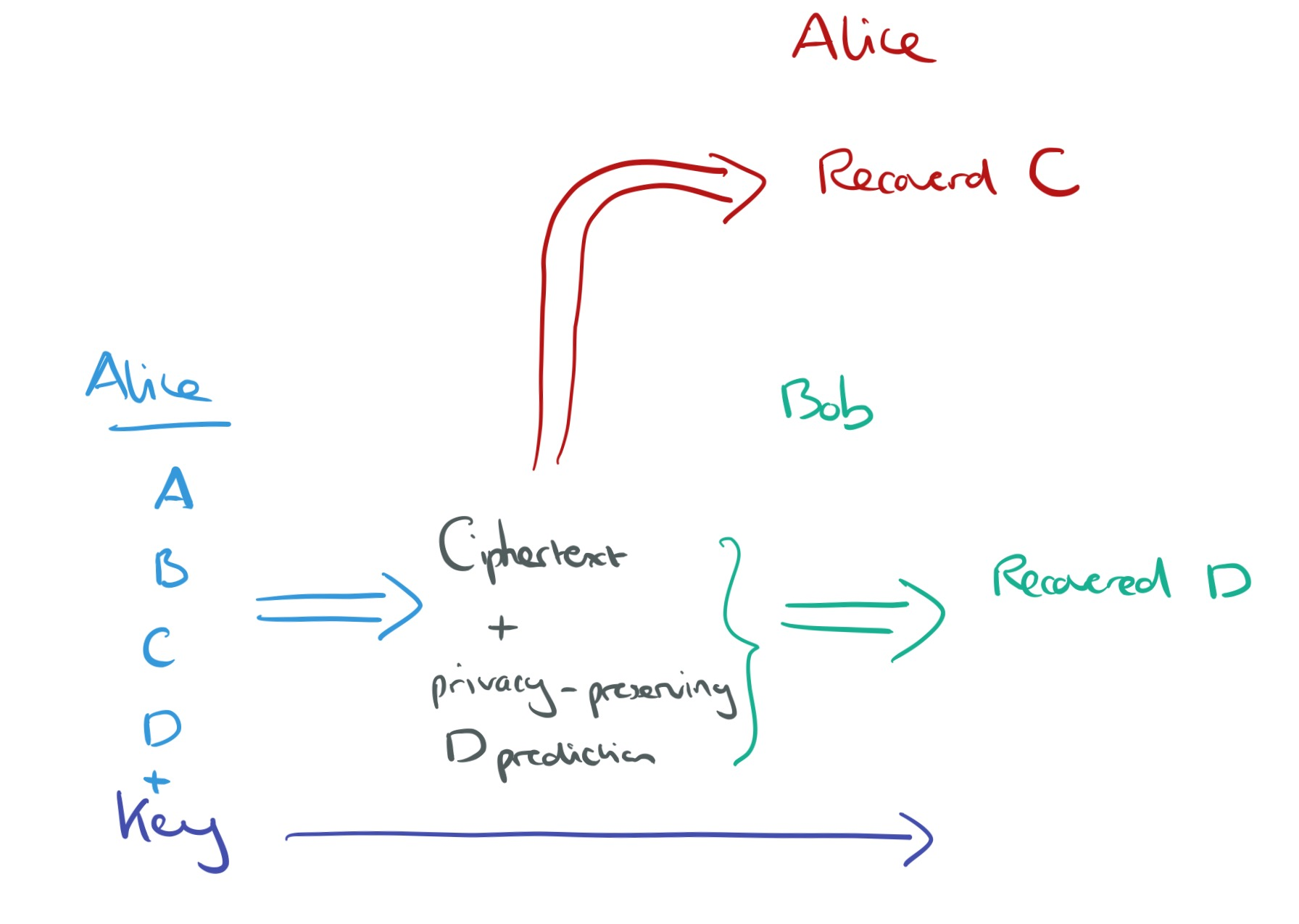… our goal is to understand whether a neural network can learn to hide information selectively, given only a high-level specification (“do not leak information about C, but let people with the right key learn as much as possible about D”).

Here are the results: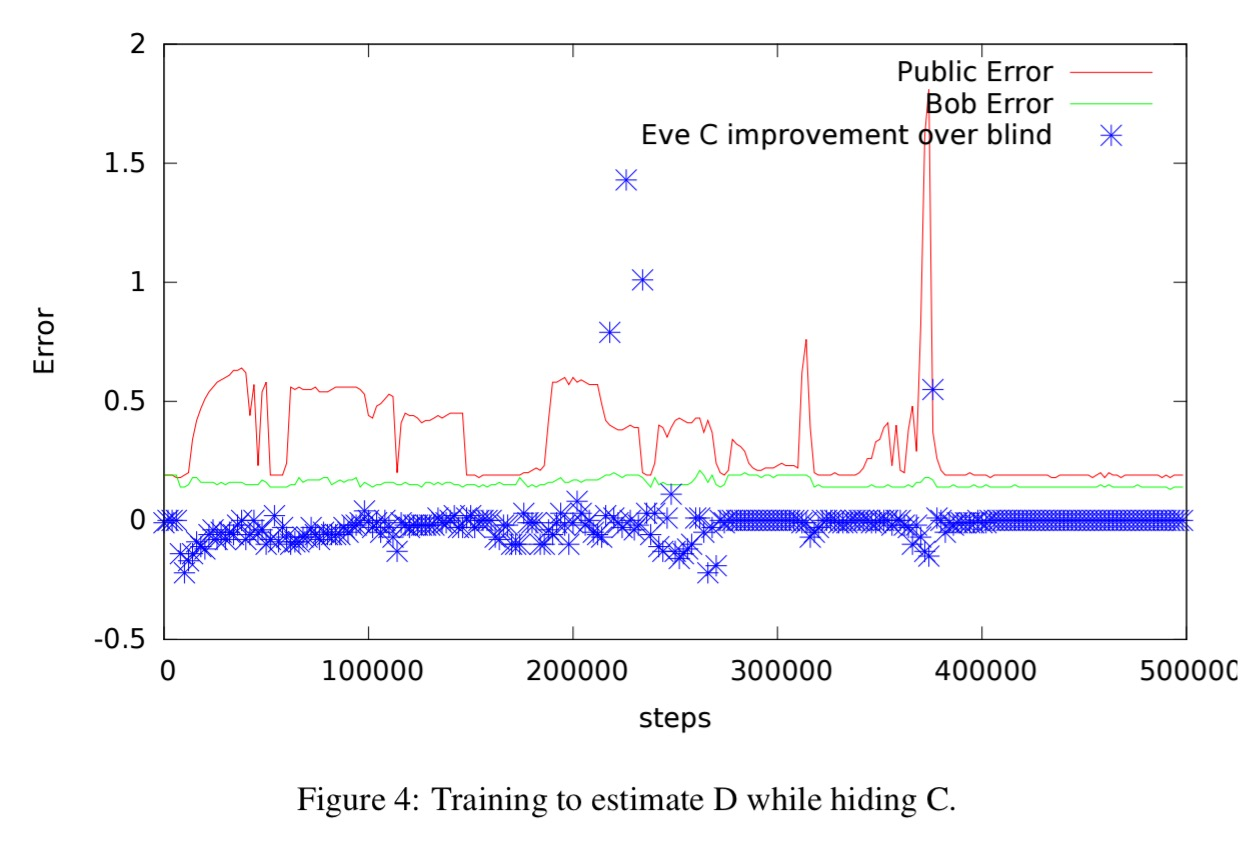The red line is the privacy-preserving prediction of D (D-public), and the green line is Bob’s attempt to recover the true value of D. The public error becomes low, but not as low as Bob’s. The blue dots show Eve’s improvement in recovering C vs blind guessing. After sufficient training, Eve can gain no more information about C than she would have by simply knowing the distribution of values of C (though that of course is still some potentially meaningful information).

Neural networks are generally not meant to be great at cryptography… Nevertheless, as we demonstrate, neural networks can learn to protect the confidentiality of their data from other neural networks: they discover forms of encryption and decryption, without being taught specific algorithms for these purposes. Knowing how to encrypt is seldom enough for security and privacy. Interestingly, neural networks can also learn what to encrypt in order to achieve a desired secrecy property while maximizing utility.

The kind of network setup shown here is a general pattern for learning goals of the form$A \wedge \neg B$ in which we want to maximise performance in task A without permitting task B to be accomplished.

In researching this work, I also found a very nice write-up with an implementation in Theano, and another implementation in TensorFlow, which makes for quite a nice comparison of the two.